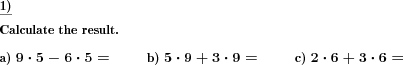Custom math worksheets at your fingertips# Details for problem "Add and subtract times table product with same factor"

Quickname: 5139

Elementary School, Primary School, Middle School.

## Summary

Addition and subtraction of times table products for a single number one to ten

## Example## Description

Practice for order of operations (PEMDAS/BODMAS rules) and times table.

This problem type trains times tables for a single number in the range from one to ten. Two products with the same second number (the fixed one) have to be added or subtracted. Only the first factor of these products differs in each product. So by adding the second product or subtracting the second product, the result moves up or down in the fixed number's times table.

As a side effect, order of execution rules are trained.

The number of problems can be adjusted. Operators can be chosen to be addition only, subtraction only or a mix of both.

Download free printable worksheets for this math problem here. The worksheet contains the problems only, the solution sheet includes the answers. Just click on the respective link.

•Worksheet 1Solution sheet with answers
•Worksheet 2Solution sheet with answers
•Worksheet 3Solution sheet with answers

If you can not see the solution sheets for download, they may be filtered out by an ad blocker that you may have installed. If this is the case, please allow ads for this page and reload the page. The solution sheets will then reappear.

• Do these sample worksheets do not really fit?
• Do you need more math worksheets, with a different level of difficulty?
• Would you like to combine different problems on a worksheet and adjust them to your needs?
• As a teacher, you can put together your own worksheets using the automatically generated math problems provided.
With a free initial credit, you can start creating your own math worksheets in a few minutes.

You can try it for free! Register here, to create custom worksheets now!

## Customization options for this problem

Parameter
Possible values
Number of problems
1, 2, 3, 4, 5, 6, 7, 8, 9, 10
Range of first factor
10, 15, 20

## Similar problems

Remark
Description
A more general approach, utilizing times tables of more numbers at the same time
Addition and subtraction of times table products